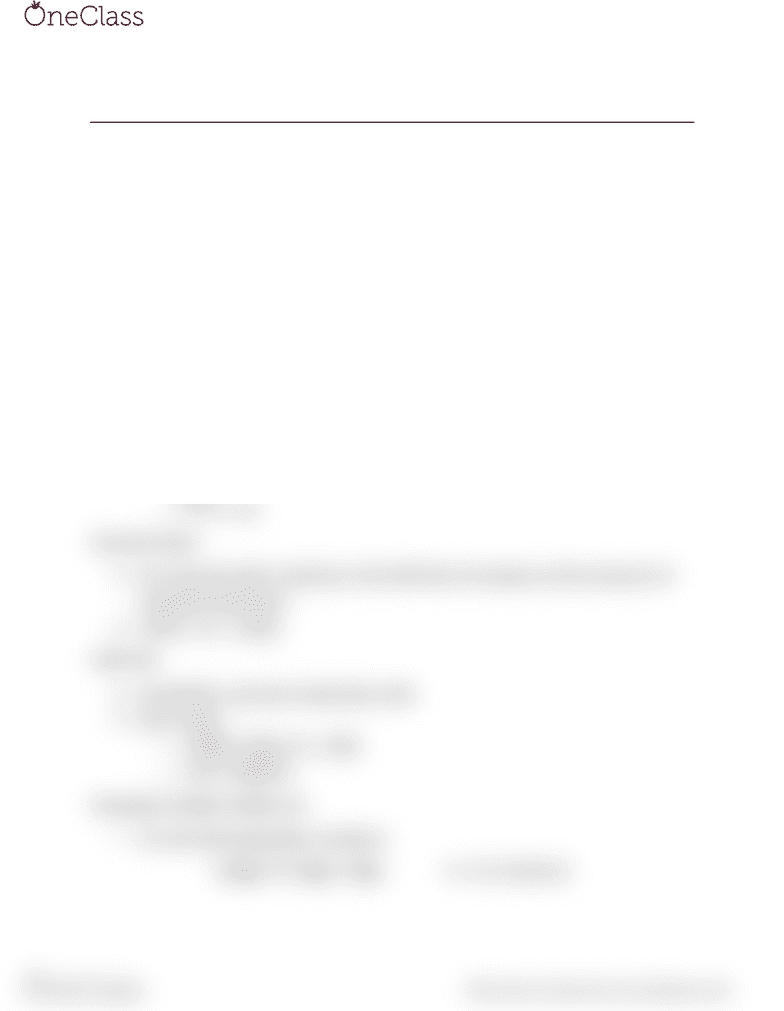# CHEM 120B Lecture Notes - Lecture 2: Rate Equation

87 views2 pagesRate Laws & Integrated Rate Laws
Integrated Rate Laws
Zeroth Order
As the reaction proceeds, the half-life becomes smaller.
Reaction Order
o The goal is to plot in such a way that the rate is
expressed/graphed linearly.
o
Y= mx + b; A0 is the concentration when t=0.
First Order
[A]t = [A]0e-kt = [A]0exp(-kt)
o Ln (At)= Ln(A0)-kt
o Y= b + mx
Second Order
For second order reactions, the half time increases as the amount of
reactants decrease.
1/[A]t = kt + 1/[A]0
Half-Life
At half life, only the initial [A] is left.
Zero order:
o ½[A0]= [At]] kt + [A0]
o t1/2= [A0]/2K
Example Problem (Slide 22)
For the decomposition reaction:
A2B(g) 2A(g) + B(g) k = 0.2 L/(mol s)
A
é
ëù
ût= - kt +A
é
ëù
û0
find more resources at oneclass.com
find more resources at oneclass.com
Unlock document

This preview shows half of the first page of the document.
Unlock all 2 pages and 3 million more documents.

## Get access

\$10 USD/m
Billed \$120 USD annuallyHomework Help
Study Guides
Textbook Solutions
Class Notes
Textbook Notes
Booster Class